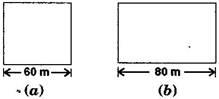# A square and a rectangular field with measurements as given in the figure have the same perimeter. Which field has a larger area?Solution

Side of a square = 60 m

Length, l = 80 m

According to the given details,

Perimeter of rectangular field = Perimeter of square field

Formula

2(l + b) = 4 × Side

2(80 + b) = 4 × 60

160 + 2b = 240

b = 40

Thus, Breadth of the rectangle is 40 m.

Area of Square field = (side)= (60)2 = 3600 m2

And Area of Rectangular field = length × breadth = 80 × 40 = 3200 m2

Hence, area of square field is larger.(18)(6)